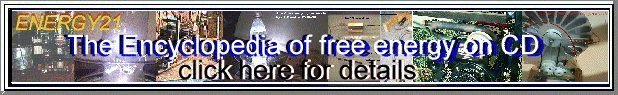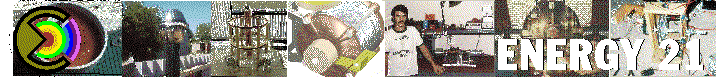PERPETUAL MOTION

BY :

E-mails:

E-mails to :

vikrantsuri@indya.com
vikrantsuri2@yahoo.co.in

 At the very outset let us make our stand very clear- we "do not" have a proposal for any "Perpetual Motion Machine" or "Free Energy Device" . We have extensively gone through web pages on Energy , Renewable Energy , Alternate Energy , New Energy etc.,etc. , and in the process came across web pages on Perpetual Motion Machines and Free Energy . As such most categorically we have nothing to do with Perpetual Motion Machines or Free Energy Devices . These terms have been used in our detailed Project Report and their usage is simply as a terminology to an expression and thus highly qualified - simply to connote a Mechanical Energy output got by a Temperature Difference ( Heat Engine - cold & hot reservoir ) created by the simple Evaporation of water - assumed as a free input for our limited use . Trust this is made amply clear - leaving no scope for mis-understanding . Admitted shortcomings of our Research work . The "concept" of our work in itself is "not" new . The concept of Vacuum-Vapour in its combination is "not" new - being known to science for long . The display of Vacuum-Vapour already exists , in many applications , and is elementary science . The Energy Balance ( Latent Heat of Evaporation of Water - small Temperature difference - Heat Engine - Laws of Thermodynamics - Result : Poor Efficiency of the models ) is and will always remain on the weaker side . Keeping this in mind - our current bigger Models weigh ( inclusive of internal Liquid contents- DiEthyl Ether - and the metallic holder/clamp ) upto 6.5 kgs ( Six and half Kilograms ) and have a height of 7 Feet ( Seven Feet ) and these are fully and efficiently functional on the Evaporation of Water - having an extractable Energy output on the Axle of movement . We feel there is cause enough for further development of this concept : with better Workshop , Tool-Room and Laboratory facilities we ourselves can improve the functional capability and Gross Output - not necessarily the efficiency - of this invention by at least a factor of ten ( 10 ) , from our current levels . At this stage a practical utility can easily come about for generating initially a small quantity of Energy / Electricity . Also keep in mind that in todays Research oriented civilisation and society - no Energy producing invention or device , as long as it is based on sound scientific principles , constructable economically and can recover ( as electricity generated ) many times over its cost of construction - should or will be ignored for long . For greater details please download from our web site ( Link - Energy Research ) Two Chapters ( about Nine Thousand words of written material ), Four Diagrams of the Models as prepared (these are original extracts from our Detailed Project Report) and some Press articles as appearing in national dailies subsequent to our Press Conference held at Chandigarh , INDIA , on 17th May 2000 , to declare and make public the worth of our invention . At our current level of work we have prepared Thirty efficient workable Models to prove the utility of the concept as also written a detailed Project Report with Fifty Seven Diagrams , explaining minutely the Models functioning and reasoning for its future potential use . For any further details or queries ( after going through part of our Project Report placed on web site www.anandgarh.org ; link Energy Research ) please contact me without hesitation and your e-mail shall be replied to within 24 (Twenty Four) Hours . Should you choose to either investigate this invention , construct your own models , ( limited workshop and toolroom facilities are required - and the models are constructable within two weeks time ) or set up a greater research leading to commercial application - please feel free to contact us for our help . Our involvement will be limited to only what you want of us . Please feel free to put forward any enquiries to me. Yours faithfully , Vikrant Suri visuri1@yahoo.com vikrantsuri@indya.com www.anandgarh.org CONTENTS ABSTRACT Section 1 : INTRODUCTION Section 2 : DEFINITIONS AND ELEMENTARY CONCEPTS Section 3 : FEASIBILITY STUDY OF CYCLIC WORK PRODUCTION FROM STEADY GRAVITATIONAL FIELDS Section 4 : FEASIBILITY STUDY OF CYCLIC WORK PRODUCTION FROM STEADY ELECTRIC FIELDS Section 5 : FEASIBILITY STUDY OF CYCLIC WORK PRODUCTION FROM STEADY MAGNETIC FIELDS REFERENCES ABSTRACT In this paper , the feasibility of cyclic work production from steady conservative force fields is to be studied through some new designs. This matter doesn't correspond with the second law of thermodynamics. Some of the designs in this paper , seem not to satisfy the second law, thus they should be considered more to find satisfying answers for them. The statement "it should be considered more" is repeated several times in this paper. It means, this paper is not a complete paper and needs more study. Some of the designs should be considered through practical experiments. Therefore, there is no conclusion for this paper before finishing the experiments. Although the violation of the second law of thermodynamics and reaching the perpetual motion seem not to be practicable, these designs may lead us to new ways of work production from sources of energy and are well worth the consideration and try. 1) INTRODUCTION Reaching the "Perpetual Motion" has been one of the oldest dreams of the man. Since long, scientists and artisans have thought about systems and mechanisms which deliver work without paying its cost. In this way, the wind and water turbines, water hammer machine and some other interesting mechanisms were invented. But in spite of all these efforts, no "Perpetual Machine" was invented to be able to produce work without spending energy or convert any types of energy to the work, in any conditions. At last, the thermodynamics put an end to all of those efforts. Nowadays, we know that for reaching the energy which is convertible to work, first of all we should pay its cost. Energy is so important to us that we have to burn the valuable black gold to use its energy with a final efficiency of below 5 percent. We can categorize various types of energy in view of their convertibility to work. Therefore the thermal energy has the lowest level so that appears in form of friction or work losses (work lost) which reduces efficiency of machines. On the contrary, electrical and hydraulical energies which can be converted to work with a high efficiency, have a high level in our classification. In this paper, we want to consider some designs in which the cyclic work is produced from "Steady Conservative Force Fields" which are known as conservers of energy. The process of production of cyclic work from steady conservative force fields doesn't correspond with "Second Law of Thermodynamics". Because of the relation between temperature of a material and its electromagnetic properties (raised from some physical phenomenons such as magnetocaloric effect or electromagnetic radiation), it may be concluded that production of cyclic work from steady conservative force fields, breaches the second law of thermodynamics. It means that the source of produced work may be only one heat reservoir with one temperature. In some of the designs of this paper, we will conclude that they are not practicable. Thus the second law of thermodynamics will be firm. But some of them seem not to correspond with the second law of thermodynamics therefore we should consider them more and more to find physical interpretations and satisfying answers for them. 2) DEFINITIONS AND ELEMENTARY CONCEPTS In this section, some key definitions and concepts which are useful to be known for this paper, are described. 2-1) PHYSICAL ANALYSIS OF CONSERVATIVE FORCE FIELDS Conservative force fields have important roles in production of work. In general, every vectorial conservative field, is a gradient of a scalar potential function. If this vectorial field has the same dimensions of the "Force", the scalar potential function will have the same dimensions of the "Energy":Where F is the force and V is a potential function. The field in which there is no disturbance, is a conservative field that the interchange of the work and energy inside it, doesn't depend on the path of a process but depends only on the primary and final positions of the process. The vectorial function "F " is the gradient of the scalar function"V ". Therefore the curl of this vector is equal to zero:The potential function of "V" satisfies Laplace's equation:2-2) THERMODYNAMICS Thermodynamics is called as "Science of Energy & Entropy". This science is based on four important laws: 2-2-1) ZEROTH LAW OF THERMODYNAMICS The zeroth law of thermodynamics is similar to the law of parallelism in geometry and states that when two bodies have equality of temperature with a third body, they in turn have equality of temperature with each other. 2-2-2) FIRST LAW OF THERMODYNAMICS The first law of thermodynamics is a particular result of the "Law of Matter and Energy Conservation" and states that a fixed amount of energy can be converted from one type to other types of energy without any decrease in quantity. This law is called "Law of Conservation of Energy".   2-2-3) SECOND LAW OF THERMODYNAMICS There are two classical statements of the second law of thermodynamics: Kelvin--Plank statement states, it is impossible to construct a device that will operate in a cycle and produce no effect other than the raising of a weight and the exchange of heat with a single reservoir. Clausius statement states, it is impossible to construct a device that operates in a cycle and produces no effect other than the transfer of heat from a cooler body to a hotter body. 2-2-4) THIRD LAW OF THERMODYNAMICS The third law of thermodynamics deals with the entropy of substances at the absolute zero of temperature and states that when the temperature is absolute zero, it has the maximum degree of order and the minimum value of entropy. 2-3) ENERGY We all know the energy. This concept raises from the first law of thermodynamics. Energy and work have the same dimensions. In fact, the energy is a capital and potentiality for work and can be converted to work through a process that satisfies both the first and second laws of thermodynamics. 2-4) ENTROPY The entropy is one of the thermodynamic properties of a system:Where, DS is the entropy difference, dQ is the heat transfer and T is the temperature of a system. This relation is a special form of the inequality of Clausius who said about the entropy in 19th Century. The more increase in entropy difference, causes more irreversibility in a process. By introducing the concept of entropy, we can conclude that between two reservoirs with temperatures of Tc and Th (temperatures of cool and hot reservoirs), the maximum efficiency of available work is the same efficiency of a Carnot cycle: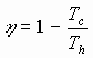The rest of this energy is not available. Since there is no reversible practical process, the energies go to be unavailable. For the first time, Kelvin said about the level of energies. Level of energy is an important physical interpretation for concept of "Total Entropy" (entropy of a system and its surroundings). Thermal energy has the lowest level in this category. The level of other types of energy goes to be lower and lower. It means that all types of energy are going to change into thermal energy as the lowest type of energy. If we liken the energy to the money in our pocket, the entropy will be the permission of spending it. For example, when we have enough money to buy a car, it means that we have a car. Because we are permitted to buy it. But, when we have enough money to buy a gun, it doesn't mean that we have it. Because we are not permitted to buy a gun. Therefore when we want to convert a type of energy to the work, we should have a process satisfying the second law of thermodynamics. The disorder or confusion is another interpretation for the entropy. When we talk about increase in the total entropy of the universe, it means that the universe is going towards disorderliness. But we believe that "Continual Creation" decreases the entropy of the universe to keep constant the total amount of entropy. 2-5) PERPETUAL - MOTION MACHINES A perpetual-motion machine of the first kind would create work from nothing or create mass-energy, thus violating the first law of thermodynamics. A perpetual-motion machine of the second kind would violate the second law of thermodynamics. A perpetual-motion machine of the third kind would have no friction and thus run indefinitely but would produce no work. 3) FEASIBILITY STUDY OF CYCLIC WORK PRODUCTION FROM STEADY GRAVITATIONAL FIELDS In this section, we want to consider some special designs in which the cyclic work may be produced from steady gravitational field. If it is practically so, the designs will not correspond with second law of thermodynamics. Thus we should consider such designs more to find satisfying answers for them. 3-1) GENERAL STUDY OF GRAVITATIONAL FIELD The gravitational field is a conservative force field. We can obtain noncyclic work from steady kind of this field or store a finite amount of energy in it potentially. In our imagination about the interaction of particles, the field acts as an intermediary. 3-2) NEWTON'S UNIVERSAL LAW OF GRAVITATION The force which two masses ( M and m with distance of r ) exert to each other, is an attractive force that is parallel with the line which connects the centers of two masses to each other and has a magnitude of:Where G is a universal constant and in the international system of units, has a magnitude of:In Newton's universal law of gravitation, the force of gravity between two particles is independent of forces or fields of other particles.Therefore the superposition method can be used for gravitational fields as conservative fields. The mathematical potential function which can define the gravitational field of a particle with mass of M is:where V is named as potential function. Since the gravitational field around a particle is spherical and symmetric, to simplify the problem, we can consider it in 2-D or 1-D mode:The main condition for V to be a potential function, is: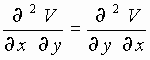The gravitational field is a sample of vectorial fields. 3-3) THE FORCE EXERTED TO A MASS ( m ) INSIDE A GRAVITATIONAL FIELD We can introduce the concept of strength of a gravitational field at a point, by force of gravity exerted to a unit of mass m placed at that point: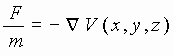Since M (maker of the main gravitational field) is a particle, the spherical coordinates can be used: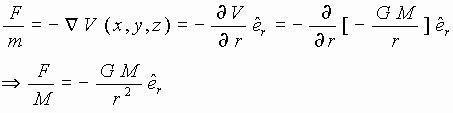Whereis a unit vector parallel with the line which connects two particles to each other. As it was mentioned before, this is the same Newton's universal law of gravitation. the negative sign, indicates that the force is attractive. 3-4) FIELD LINES AND POTENTIAL LINES IN GRAVITATIONAL FIELD In 2-D mode study of the gravitational field, since the attractive forces of gravity are centripetal, the field lines (or force lines) are lines which converge at the particle M.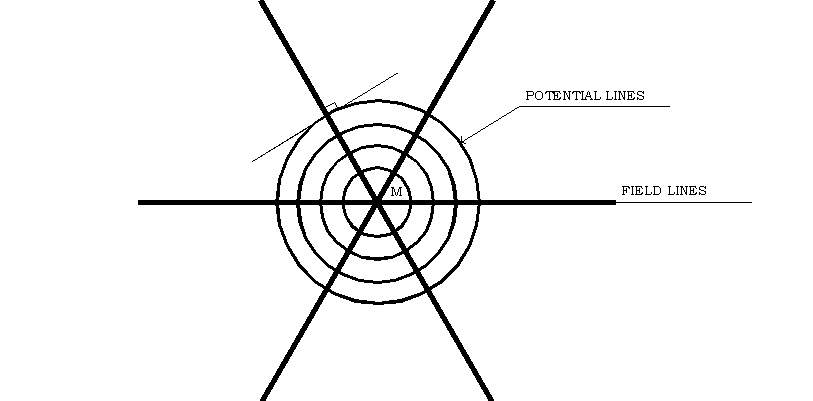Fig. 3-1) Field lines and potential lines of a gravitational field The potential lines are perpendicular to the field lines. Thus the potential lines are concentric circles which the particle M is their center. 3-5) LAW OF CONSERVATION OF ENERGY IN GRAVITATIONAL FIELD Consider two points of A and B in a gravitational field. Since the change in the potential energy of a particle M in the field is independent upon displacement path in the field, we can write: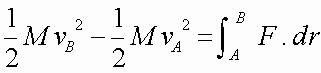Where v is velocity of M,is kinetic energy of M, and result of the left part of the equation is the variation of kinetic energy through displacement of M from point A to point B. The result of integral is the work done on particle M through process of displacement. Therefore the law of work and kinetic energy is defined as: "The difference in kinetic energy is equal to the done work". So we can write: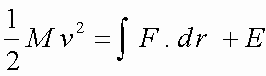In which, E is a constant for the integral.In the conservative force fields, V is the potential energy and F is the conservative force. The following equation which is called "Integral of Energy" , states that sum of kinetic and kinematic energies of a particle is constant along path of displacement in a conservative force field: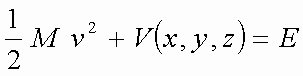3-6) PRODUCTION OF CYCLIC WORK FROM STEADY GRAVITATIONAL FIELDS In this section we want to consider feasibility of production of cyclic work from steady gravitational fields. This doesn't correspond with second law of thermodynamics. In some of the following designs, we are able to prove that the second law of thermodynamics is valid for them. But some of them seem not to satisfy the second law and should be considered more to find satisfying answers for the unsolved problems in this section. 3-6-1) MOVEMENT OF A PARTICLE IN A GRAVITATIONAL FIELD As a false assumption, suppose that we can have a space around a particle in which we are able to replace the field and potential lines with each other. If so, the field lines will be concentric circles . Of course, this assumption has no physical or mathematical interpretation and is incorrect and false. Now, if the particle M moves from point A to point B inside this imaginary field, according to the law of conservation of energy, it will produce work.Fig. 3-2) Sketch for design (3-6-1) To continue the process, particle moves from point B to point A (in the same direction) and completes one cycle. In this cycle, the particle moves through a circular path and returns to its initial position A. Thus it will be able to do the same in the next cycle. Consequently in this imaginary gravitational field, we can obtain work cyclically and uniformly. This matter doesn't correspond with first or second law of thermodynamics. By sketching out this imaginary process, we want to point to the closed circuitous form of field lines in a magnetic field as a conservative field and feasibility of production of cyclic work from steady kind of this field in the section 5 . Now consider the actual form of field lines in a gravitational field (for example gravitational field of the earth). We know that there is no insulator for a gravitational field i.e. nothing has been found as an insulator for it until now, to be able to prevent the flow of its lines. But we want to know whether a fluid with exerting the "Buoyant Force" to a submerged body inside it, can have role of an insulator which reduces force of gravity of the body. The following design answers: In figure (3-3), there is a liquid with density of r 2 inside a pool. A solid cube with dimensions of a * a * a and density of r 1 can pass the sealed up gates of G 1 and G 2 without penetration of liquid.Fig. 3-3) 2nd sketch for design (3-6-1) The produced and spent works in this process can be calculated. We know that if the cube does not enter the pool, the net produced work in a closed path inside the conservative gravitational field will be equal to zero, because of independence of net produced work upon the path. But we want to know whether the buoyant force of the liquid is able to affect the net produced work. In other words, are we able to return an elevated mass to the same height by the buoyant force after obtaining work from its potential energy to complete the cycle ? If so, we will be able to produce cyclic work from steady gravitational field as a conservative force field. Calculations: When the cube moves from position A to position B along the path AB :In the path BC, to cause the cube to pass the gate G 1, we have to do work (spend energy) to overcome the force produced from hydrostatic pressure of liquid on the surface of the cube:In the path CD, through a quasi-static process, the buoyant force on the cube, not only can elevate it and increase its potential energy, but also produces work. To simplify the calculations, we can prevent flotation of cube on surface of liquid. So we can write: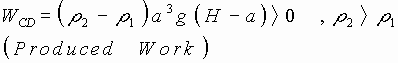In the path DA, the cube returns to initial position A, through passing the gate G 2 and completes the cycle: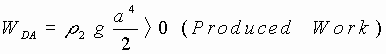In this design, we assume a very large surface for pool to be able to ignore variation in level of fluid in it, when the cube enters the pool. Of course, this variation in level has no effect on the result. For the net work production we can write:This shows that we can not assume a fluid and its buoyant force on submerged bodies inside it, as an insulator for the gravitational field. In fact, we can return the cube to its initial level by buoyant force, but to cause the cube to enter the container of fluid at the lower level, we have to spend all the produced work through the cycle. The amount of this spent work is equal to product of multiplication of the volume of cube by the hydrostatic pressure of the fluid at depth of ( H - a /2) inside the pool (level of geometric center of cube). Here, as a case study, we can consider another way to enter the cube into the pool of liquid. In this way, before entering the cube into the pool, we can cool it to reduce its volume and consequently the work done for this step of the cycle. In this study, the net produced work depends upon the coefficient of volume expansivity (and its effect on geometric center of the cube), heat capacity, effect of the hydrostatic pressure of the water of pool on the volume and temperature of cube and so on. If the produced work is not equal to zero, this problem will not correspond with the second law of thermodynamics. This problem is well effort to be considered. 3-6-2) MOVEMENT OF A BALLOON INSIDE A FLUID Movement of a balloon in a fluid with more density than that of the balloon, satisfies first and second laws of thermodynamics the both. But we want to consider another balloon with some singular properties: As we well know, water at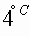is the densest water. When we cool the water up to(triple point), it changes into ice (solid state) with a lower density. Now we can consider a special balloon with water as the "Working Fluid" and submerged inside an ocean of water at. This ocean has role of a constant high-temperature reservoir and its surroundings has role of a constant low-temperature reservoir with temperature of(or less than it). The reservoirs are isolated from each other by insulator. In an ordinary balloon, we should warm its working fluid to reduce its density. But in this singular balloon, we have to cool its working fluid (the water inside the balloon) to reduce its density. In figure (3-4), consider the cycle A-B-A. At the position A, the temperature of water inside the balloon (working fluid) is. Therefore, the water of ocean doesn't exert any buoyant force to the balloon.Fig. 3-4) Sketch for design (3-6-2) To reduce the temperature of water (and its density), we should transfer its heat to a low temperature reservoir. This heat transference can produce work by means of a heat engine. Because of limitation of the heat capacity of the balloon (with insulated walls), it can't be assumed as a constant-temperature reservoir. Thus the efficiency of this heat engine will be less than efficiency of a heat engine operating between two fixed temperature reservoirs. When the temperature of working fluid of the balloon reaches, the buoyant force lifts it. Along the path A-B the balloon can produce work. At the position B we should increase temperature of working fluid of the balloon to increase its density to be able to return it to the position A without spending any energy (through a quasi-static movement with no viscose friction). Therefore in the position B, heat is transferred to the balloon through a heat engine with an efficiency less than that of a Carnot cycle, because of limitation of the heat capacity of the working fluid of balloon. Returning to position A, the cycle will be completed. In this design, we have work production in three steps of the process. The balloon and heat engine, are two intermediaries between two constant-temperature reservoirs. Assuming the balloon and its heat engine as a machine operating between two reservoirs (ocean and its surroundings), we want to know whether the operating efficiency of this machine can exceed efficiency of a Carnot cycle. This problem should be considered more. In this study, we should consider some factors such as effect of hydrostatic pressure of ocean on the triple point, mean Carnot efficiency of the heat engine operating between two temperatures when one of them is not constant, difference of density between water and ice, amount of vertical displacement of balloon in the ocean and so on. 4) FEASIBILITY STUDY OF CYCLIC WORK PRODUCTION FROM STEADY ELECTRIC FIELDS   In this section, we want to consider some designs in which the cyclic work may be produced from arrangements of steady electric fields. If it is practically so, the designs will not correspond with second law of thermodynamics. Thus we should consider such designs more to find satisfying answers for them. 4-1) GENERAL STUDY OF ELECTRIC FIELD First of all, we should know about the "Electric Charges" to be able to study electric fields as an intermediary between electric charges. There are two kinds of electric charges, positive and negative charges. The electric charges are not created and do not vanish. this is called as "Law of Electric Charge Conservation". As it is shown in figure (4-1), a line a tangent to which at every point coincides with the direction of the field strength is called a "Line of Force" of the electric field or a "Field Line". The lines perpendicular to the field lines are called "Equipotential Lines", on which, all the points have the same potential. The potential at a given point of a field is a "Scalar Quantity" numerically equal to the potential energy of a unit positive chargeat this point. The potential difference between two points of an electric field is called "Voltage" and is numerically equal to the work done by electric forces through moving a unit positive charge between two points.Fig. 4-1) Field lines and equipotential lines   The electric field is similar to the gravitational field:Where E is magnitude of the electric field. The law of interaction of the point charges ( "Coulomb's Law") in an inertial reference frame, relative to which they are stationary is written as follow:Thus the electric field strength of a point charge is: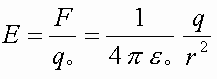The method of superposition is valid for the electric fields:Coulomb's law can be stated in another form which is called "Gauss's law". This law is used for any bounded surface and is a relation between the flux passing the bounded surface F and the absolute charge q inside it:Where dS is the surface element and K is the "Relative Permittivity". 4-2) MATHEMATICAL ANALYSIS OF ELECTRIC FIELD In this section, to simplify this analysis, we can consider the problem in 2-D or 1-D mode because of symmetry of the fields:The main condition for V to be a potential function, is: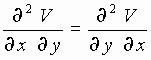The force exerted to the unit positive charge in the electric field induced by the charge q is (1-D mode):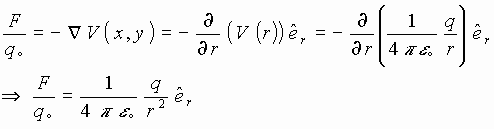This is the same Coulomb's law. 4-3) SOME SPECIAL PROPERTIES OF ELECTRIC FIELDS The electric field has some special properties. For example there are natural electrical insulating materials for this kind of force fields. These insulators are called "Dielectric". The gravitational and magnetic fields have no insulator all around the world. In section 3 we saw that when a fluid exerts buoyant force to a submerged body inside it and reduces its weight, the fluid can't be assumed as an insulator. For example, the buoyant force can submerge a submarine in the water but is not able to reduce the weight of members of crew. Since there are two kinds of charges in the electricity, the electric charges can exert attractive and repellent forces to each other and also, unlike charges can make electric dipoles. In the gravitation, there are not any unlike particles. Thus the gravitational forces are only attractive and the gravitational dipole can not exist. In the magnetism, there is no singular magnetic element and the magnetic dipole is the most basic element and magnetic monopole can not exist. According to above special properties of the electric fields, we want to consider whether it is possible to produce cyclic work from steady electric fields. If so, the perpetual-motion machine of the second kind would be made. 4-4) PRODUCTION OF CYCLIC WORK FROM STEADY ELECTRIC FIELDS In this section, we want to consider feasibility of production of cyclic work from steady electric fields. This doesn't correspond with second law of thermodynamics. In some of the following designs, we are able to prove that, the second law is valid for them, but some of them seem not to satisfy the second law and should be considered more. 4-4-1) MOVEMENT OF A POINT ELECTRIC CHARGE IN AN ELECTRIC FIELD In this problem, we want to consider production of work through movement of a charge inside an electric field and whether this work is cyclic. In figure (4-2), a charge passes through a closed path in an electrostatic field. In one cycle, it starts from a point and returns to the same point again. It means, the charge goes from a potential to the same potential and therefore produces no work through one cycle.Fig. 4-2) Sketch for design (4-4-1) Therefore the second law of thermodynamics is valid for this problem. 4-4-2) LIMITATION OF AN ELECTRIC FIELD IN A BOUNDED SPACE Figure (4-3) shows a bounded electric field which a point charge q moves inside it through the path 1-2-3-4-1. In the path 1-2 the electric charge produces work. Now we want to know whether it is possible to return the charge to its initial position (1) without spending any energy. To reach this purpose, we need a bounded electric field. Apart from our ability in making such a field, movement of a charge outside a bounded field, spends no work.Fig. 4-3) Sketch for design (4-4-2) Since the paths 2-3 and 4-1 are equipotential and the path 3-1 is outside the field , the charge spends no work through the path 2-3-4-1 to move. Therefore in the cycle 1-2-3-4-1 the charge can produce cyclic work. If possible, it doesn't correspond with second law of thermodynamics. Thus the ability of making such a field (bounded electric field) must be considered. Hence the role of insulators in the electric fields should be studied. 4-4-3) ELECTRIC FIELD INDUCTED BY A UNIFORMLY CHARGED LARGE PLATE Figure (4-4) shows a large, thin and uniformly charged plate which inducts a homogeneous electrostatic field that the direction of the field changes inversely at the plate. We want to know whether this inversion in the direction of the field, causes to produce cyclic work from this steady conservative force field. Hence, we study the movement of an electric dipole inside this field.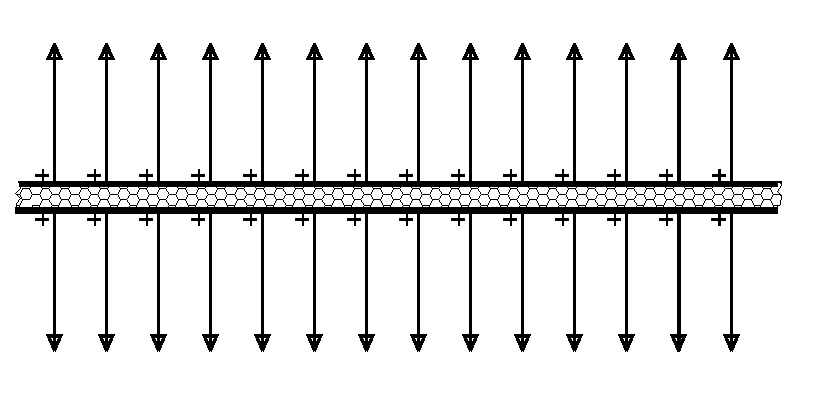Fig. 4-4) Sketch for design (4-4-3) The homogeneous electric field exerts a couple to an electric dipole, so that the dipole is able to rotate around any centers. In this problem, the electric dipole is jointed at the point O as a rotating joint and center of rotation, to be able to rotate around it and complete the cycle 1-2-3-4-1.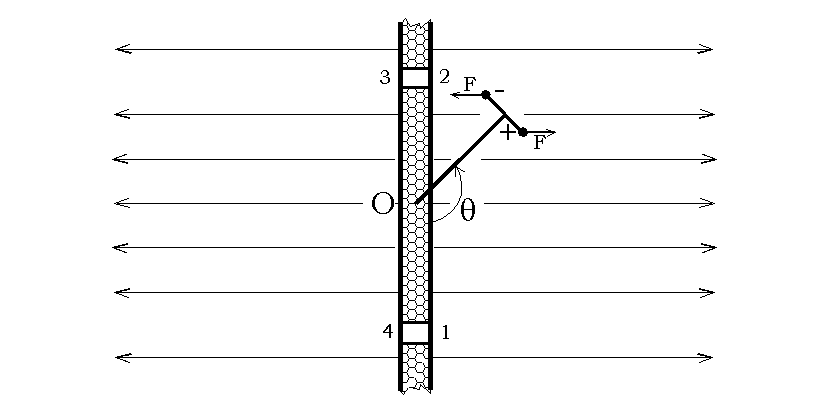Fig. 4-5) 2nd sketch for design (4-4-3) In the path 1-2 the dipole rotates a half round and produces work (like the path 3-4). But in the paths 2-3 and 4-1, the dipole can pass through small holes on the plate and consumes energy during this pass. Considering this design, we have: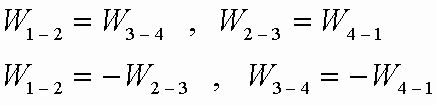Where W is the work produced or spent in every path, corresponding with its index. Therefore we can conclude that:It means that the cyclic work can not be produced through this design. 4-5) UTILIZING DIELECTRIC INSULATORS IN THE DESIGNS In the previous designs, the produced and spent works were equal to each other in a cycle. Now we want to consider whether we are able to use dielectric insulators to insulate the returning paths in which the work is spent and consequently reduce the force exerted to the moving charge in the returning paths or bound the electric field by the insulators. If so, the produced work will be more than the spent work in a cycle and it will be possible to produce cyclic work from steady electric fields as conservative force fields. The following problem indicates: Here, we study the effect of a dielectric insulator on the force between two parallel plates of a capacitor. Figure (4-6) shows a capacitor with parallel plates and without any dielectric insulator. The capacitance of this capacitor is:Where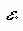is the permittivity of the vacuum, A is the area of the plates and d is the distance between the plates. In fact, C is the ratio of the charge on one of the plates divided by the potential difference between them: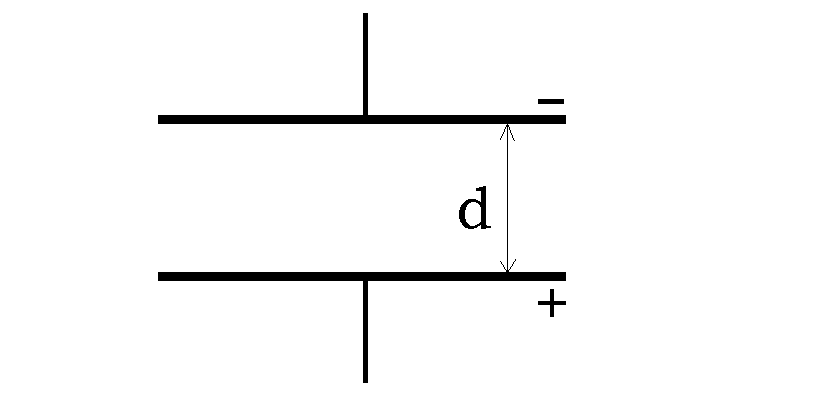Fig. 4-6) Capacitor without dielectric insulator If the plates are connected to a battery with a potential difference of V and then are disconnected from it, the stored energy in the capacitor will be:Because of disconnection of the capacitor from battery, q is constant during our consideration. To calculate the interaction of the plates of a capacitor, first of all we keep them at a more distance of x so that the total distance between two plates becomes d + x (fig. (4-7)). Thus the new capacitance will be:Since the force between the plates is an attractive force, when their distance increases, the energy stored in the new capacitor (with more capacitance) will increase too:Consequently: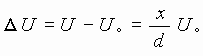Fig. 4-7) Displacement of a plate of the capacitor If the displacement x is too small, it can be written:Where F is the force which the plates exert to each other. Now consider the same capacitor with a cake of dielectric insulator between its plates (fig. (4-8)). So: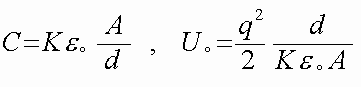Where K is the relative permittivity of the insulator.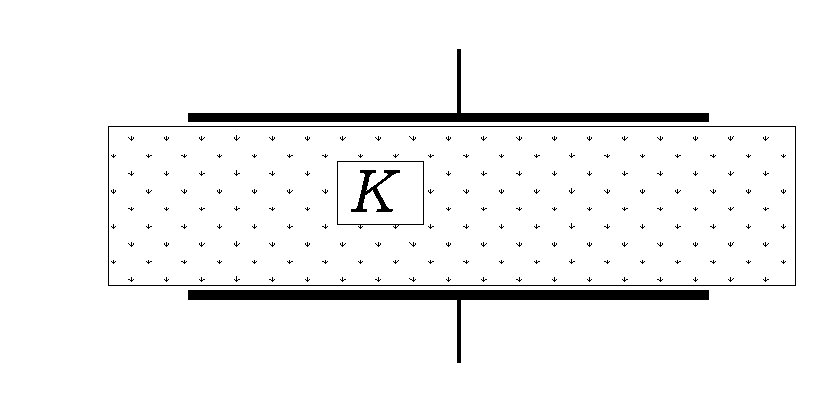Fig. 4-8) Capacitor with dielectric insulator In figure (4-9), the distance between two plates increases.Fig. 4-9) Displacement of a plate of the insulated capacitor Thus:If x is too small, we can write: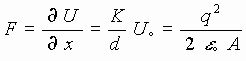This conclusion shows that the force between plates of a capacitor without dielectric insulator is equal to the force between plates of a capacitor with dielectric insulator. This means that: "The dielectric insulators have no effect on interaction of electric unlike charges". The effect of insulators on interaction of like charges should be considered and experienced. 4-6) ELECTRIC FIELDS CREATED BY ALTERNATED MAGNETIC FIELDS The alternated magnetic fields can make closed electric field lines which are shown in figure (4-10).Fig. 4-10) Closed electric field lines By this special electric field, we are able to produce cyclic work. But this process doesn't violate the second law of thermodynamics, because this special electric field is not a conservative force field and needs a permanent source of energy. 5) FEASIBILITY STUDY OF CYCLIC WORK PRODUCTION FROM STEADY MAGNETIC FIELDS In this section, we want to consider some special designs in which the cyclic work may be produced from arrangements of steady magnetic fields. If it is practically so, the designs will not correspond with the second law of thermodynamics. Thus we should consider such designs more to find satisfying answers for them. 5-1) GENERAL STUDY OF MAGNETIC FIELD The "Current-Carrying Conductors" and "Magnets" interact with one another. This interaction occurs by means of what we call it "Magnetic Field". A magnetic field is detected by its action on moving charges or "Magnetic Needles". The vector quantity B, known as the "Magnetic Induction" is introduced to characterize a magnetic field. The direction of the magnetic induction vector coincides with that of the force acting on the north end of a magnetic needle placed at the given point in the field. The force acting on a current-carrying conductor, placed in a magnetic field is determined by "Ampere's Law":Where i is the current, Dl is the element of length of the conductor. The SI unit for the induction is tesla ( T ). 1T is the magnetic induction of a field acting on a unit current element (i.Dl) of 1A.m at right angles to the induction vector with a force of 1N. A quantity called the "Magnetic Field Strength", H (previously known as the magnetic field intensity) is used in addition to the magnetic induction B. The magnetic field strength in a vacuum is defined to be the quantity equal to the ratio of magnetic induction B to the "Magnetic Constant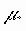" :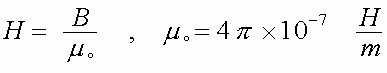In general:In which,is the "Relative Permeability" and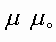is called the "Absolute Permeability" of a medium. In an isotropic medium, the vector B and H have the same direction. The direction of the force acting on a current-carrying conductor is determined by the "Left-Hand Rule": If the palm of the left hand is placed so that the magnetic field lines enter it and the extended fingers show the direction of the current, the thumb will indicate the direction of the force acting on a conductor. 5-2) SOME SPECIAL PROPERTIES OF THE MAGNETIC FIELD The magnetic field has some special properties which may be useful in our study about feasibility of cyclic work production from steady magnetic fields: Magnetic field has closed field lines. The most simple element in the magnetism is the magnetic dipole and there is no magnetic monopole at all. There is no natural insulator for the magnetic field. 5-3) PRODUCTION OF CYCLIC WORK FROM STEADY MAGNETIC FIELDS In this section, we want to consider feasibility of production of cyclic work from steady magnetic fields. This doesn't correspond with the second law of thermodynamics. In some of the following designs, we are able to prove that the second law is valid for them, but some of them seem not to satisfy the second law and are well worth the effort a try and more consideration. 5-3-1) MAGNETIC FIELD AROUND A LONG STRAIGHT CURRENT-CARRYING CONDUCTOR Figure (5-1) shows that the magnetic lines around a straight and long conductor with a linear electric current, are concentric circles (2-D mode). All the magnetic lines are closed lines. Here, we want to know whether we are able to use this special property to produce cyclic work from such a steady magnetic field.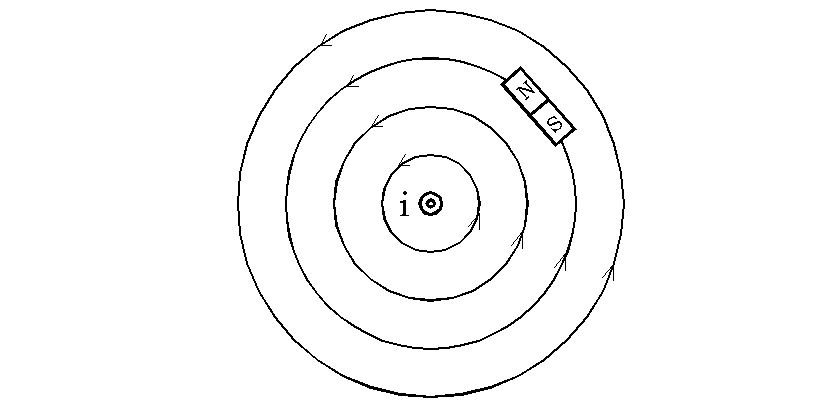Fig. 5-1) Magnetic field lines around a linear current When a magnetic dipole is located in a magnetic field, a moment is exerted to it to conform its direction to that of the magnetic field. Therefore a magnetic dipole inside a concentric field will be directed along a tangent to the circles (or curves) in any points in the field. In figure (5-2), a magnetic dipole is located in a perpendicular position to the magnetic lines which are concentric circles and is provided with a rotating joint that its center of rotation is the same center of magnetic circular lines: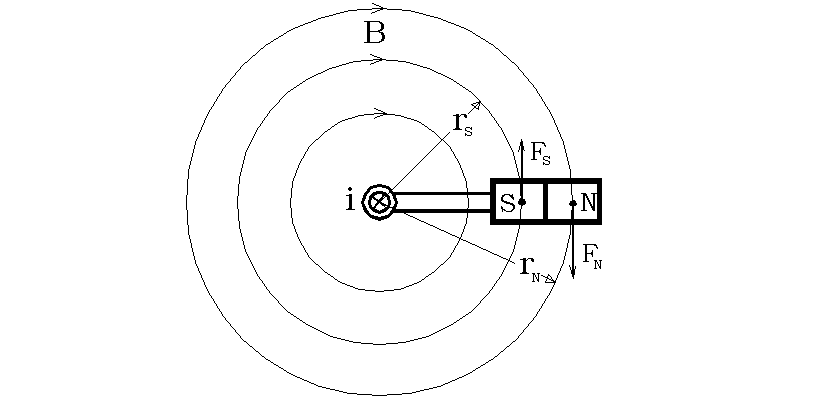Fig. 5-2) Sketch for design (5-3-1) In this situation, two moments are exerted to the dipole. To calculate the net moment, we should calculate two moments exerted to the poles:K is a constant.This design shows that, if we are able to make a magnetic field with concentric circle (closed) lines in which the magnitude of B is not proportional to (1 / r), we will be able to make a perpetual-motion machine of the second kind. It should be noted that the rotation of magnetic dipole in such conditions doesn't induct any current into the conductor i.e. no counter electro-motive force is inducted into the conductor. See the next design. 5-3-2) MAGNETIC FIELD INSIDE A CURRENT-CARRYING CONDUCTOR In figure (5-3), the shown magnetic dipole is provided with a rotating joint with the same center of the normal section of conductor. The conductor might be a cylindrical container which contains liquid conductor. Therefore the magnetic dipole can be immersed inside the liquid conductor and rotate. This design is similar to the design (5-3-1) but here, the magnitude of B is not proportional to (1 / r) thus the net moment exerted to the dipole might be more than zero.Fig. 5-3) Magnetic induction inside and outside of a current-carrying conductorThe achievement of success in this design, should be proved through practical experiments. 5-3-3) MAGNETIC FIELD CREATED BY A PIPELIKE CURRENT-CARRYING CONDUCTOR A pipelike conductor carrying a steady electric current is shown in figure (5-4). The pipe has a gap with flexible conductive brush, through which a magnetic dipole can pass. Movement of the dipole through the flexible conductive brush doesn't cause production of counter electromotive force, because the current of the pipe passes through a path, perpendicular to the field lines and crosses over them two times in different directions of the field lines. According to Ampere's law, no magnetic field exists in inner space of the pipe. The magnetic lines outside the pipe are concentric circles and the outer half of the magnetic dipole is located in the magnetic field outside the pipe. Therefore this magnetic field exerts a force to the pole which is located in the outer side of the pipe.Fig. 5-4) Sketch for design (5-3-3) Since the dipole is provided with a rotating joint located on the central axis of the pipe, this force becomes a moment which is able to turn the magnetic dipole. If it is so, the cyclic work can be produced from steady magnetic fields and it will not correspond with second law of thermodynamics and needs to be considered more. 5-3-4) MAGNETIC FIELD CREATED BY A LONG PLATE CARRYING A STEADY CURRENT Figure (5-5) shows normal cut of a large plate which carries a steady electric current. The magnetic field inducted by the current, is a homogeneous magnetic field which has different directions in different sides of the plate.Fig. 5-5) Sketch for design (5-3-4) Now, consider a cycle in which a magnetic dipole moves through the path 1- 2-3-4-1 in this field. In the path 1-2 , the dipole turns a half round and the produced work in this step is equal to the product of B multiplied by "Magnetic Dipole Moment" ( P): W = P.B (2 p ) > 0 In the path 2-3, the dipole moves in a parallel status with the field lines. Thus no couple is exerted to it. The path 3-4 looks like the path 1- 2 and the work W is produced. The path 4-3 looks like the path 2-3 and work is produced through it. The net produced work can be calculated as the following relation:This means, the net produced work through this cycle exceeds zero. If it is so, it will not correspond with second law of thermodynamics and we should consider it more to find a satisfying answer for it. 5-3-5) MAGNETS WITH OBLIQUE FIELD LINES Figure (5-6) shows an arrangement of a large number of simple magnetic dipoles (magnetic needles) which are arranged obliquely beside each other.Fig. 5-6) Sketch for design (5-3-5) In this situation, the magnetic lines are parallel lines perpendicular to the line which connects the middle of the magnetic dipoles, because in this arrangement of the dipoles, the magnetic lines connect the opposite poles through the shortest paths. Figure (5-7) shows the same arrangement of the magnetic dipoles with imaginary magnetic lines which are oblique. If we are able to create such a magnetic field, making a perpetual-motion machine of second kind will be practicable.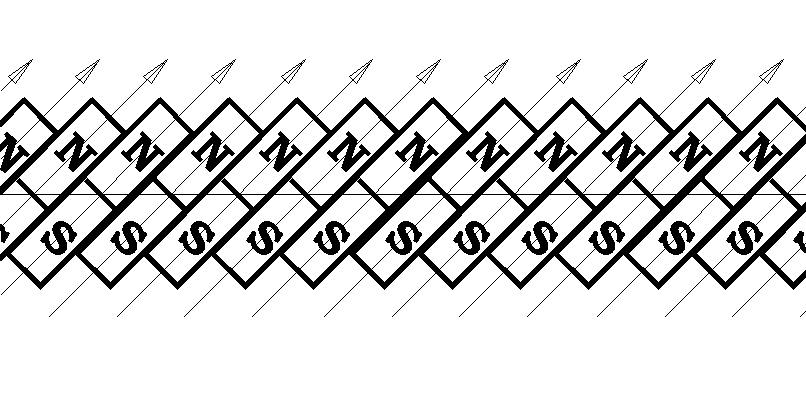Fig. 5-7) 2nd sketch for design (5-3-5) Therefore the ways to make such an oblique magnetic field should be considered more, through experiments. 5-3-6) FLUID FERROMAGNETIC SUBSTANCE If there might be any fluid ferromagnetic substances, a magnetic dipole would be able to pass a suitable path inside the fluid ferromagnetic substance and produce cyclic work from the conservative force field. If so, it will not correspond with second law of thermodynamics and should be considered more. 5-3-7) MAGNETIC FIELD AROUND A TOROID Here, we want to study the magnetic field around a toroid. As we well know, there is no magnetic field around a toroid in theory and all of the magnetic lines pass through the ferromagnetic ambient as a core. But a very weak magnetic field, appears around the toroid in practice. If it is so, the perpetual motion will be practicable because, there are some sketches to use these diffused magnetic field lines to produce cyclic work. This matter should be considered more through practical experiments.  REFERENCES 1) N. Moniri , " Thermodynamics and Heat " , 2nd Edition , Tehran University Publications, 1999. 2) M. N. Bahrami , " Vectorial Dynamics " , Tehran University Publications , 1994. 3) A. Feyz Dizaji , " Advanced Mathematics for Engineering and Physics " , Tehran University Publications , 1994. 4) N. I. Koshkin , M. G. Shirkevich , " Handbook of Elementary Physics ", Mir Publishers , (Translated from Russian by G. Leib) , 1982. 5) E. Kreyszig , " Advanced Engineering Mathematics " , John Wiley & Sons , 1979. 6) D. Halliday , R. Resnik , " Physics (Vol. 1, 2 , 3) " , John Wiley & Sons , 1978. 7) M. W. Zemansky , R. H. Dittman , " Heat and Thermodynamics " , Mc Graw-Hill , 1981. 8) G. J. Van Wylen , R. E. Sonntag , " Fundamentals of Classical Thermodynamics " , John Wiley & Sons , 1985. 9) F. P. Incropera , D. P. Dewitt , " Fundamentals of Heat and Mass Transfer " , John wiley & Sons , 1990.  HAMID REZA SAMADINIA SHALMAEE E-mails: hrss@abdnet.com h.r.samadinia@ikco.com hrss2@yahoo.comWeb www.IceStuff.com